# Engineering Mechanics - PKRB: Force and Animation

### Exercise :: PKRB: Force and Animation - General Questions

• PKRB: Force and Animation - General Questions
11.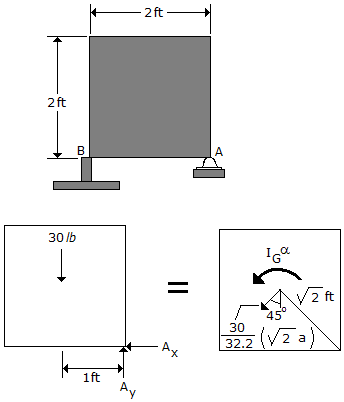If the support at B is suddenly removed, determine the initial reactions at the pin A. The plate has a weight of 30 lb.

 A. Ax = 22.5 lb, Ay = 7.5 lb B. Ax = 0, Ay = 30.0 lb C. Ax = 11.25 lb, Ay = 18.75 lb D. Ax = 11.25 lb, Ay = 41.3 lb

Explanation:

No answer description available for this question. Let us discuss.

12.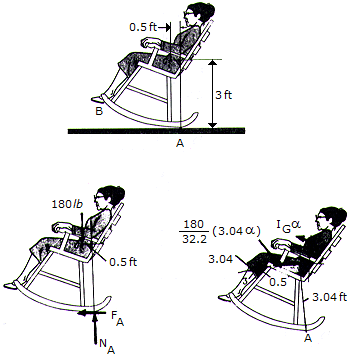A woman sits in a rigid position on her rocking chair by keeping her feet on the bottom rungs at B. At the instant shown, she has reached an extreme backward position and has zero angular velocity. Determine her forward angular acceleration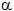and the frictional force at A necessary to prevent the rocker from slipping. The woman and the rocker have a combined weight of 180 lb and a raduis of gyration about G of kG = 2.2 ft.

 A.= 1.185 rad/s2, F = 19.87 lb B.= 1.143 rad/s2, F = 19.16 lb C.= 1.163 rad/s2, F = 19.51 lb D.= 3.16 rad/s2, F = 5301 lb

Explanation:

No answer description available for this question. Let us discuss.

13.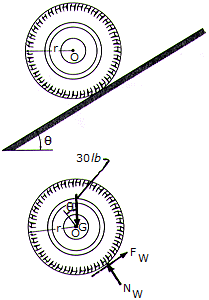The wheel has a weight of 30 lb, a radius of r = 0.5 ft, and a radius of gyration of kG = 0.23 ft. If the coefficient if friction between the wheel and the plane is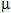= 0.2, determine the wheel's angular acceleration as it rolls down the incline. Set= 12°.

 A.= 59.5 rad/s2 B.= 8.93 rad/s2 C.= 11.05 rad/s2 D.= 63.3 rad/s2